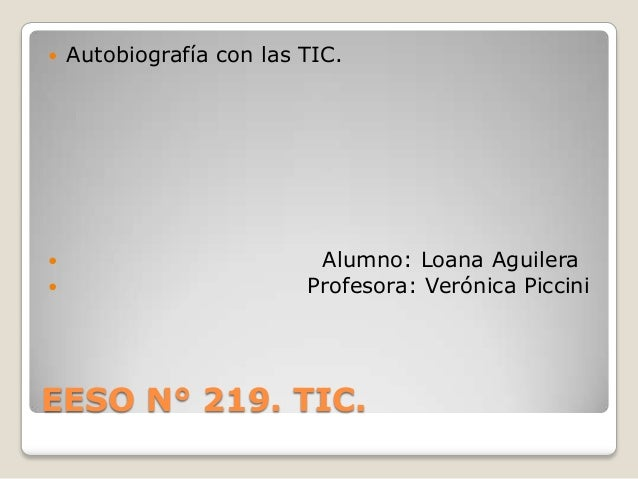Successfully reported this slideshow.Upcoming SlideShare
×

# Trabajo de "autobiografía con las tic" EESO N° 219.

Trabajo de nuestra autobiografía con las tic, que evolucionaron a nuestro alrededor. En la Escuela de Enseñanza Secundaria Orientada N° 219.

• Full Name
Comment goes here.

Are you sure you want to Yes No• Be the first to like this

### Trabajo de "autobiografía con las tic" EESO N° 219.

1. 1. EESO N° 219. TIC.  Autobiografía con las TIC.  Alumno: Loana Aguilera  Profesora: Verónica Piccini
2. 2. N a c i e l 23 d e f e b r e r o d e 1996
3. 3. Mi primer teléfono.
4. 4. 2 .
5. 5. 3
6. 6. 4
7. 7. 5
8. 8. 6
9. 9. 7
10. 10. 8
11. 11. 9
12. 12. 10
13. 13. 11
14. 14. 12°  R e e m p l a z a r o n a m i B l a c k B e r r y , m i e n t r a s e s t a b a e n a r r e g l o .
15. 15. N u e v a m e n t e mi B l a c k B e r r y .
16. 16. M i t e l e v i s o r
17. 17. N o s a c t u a l i z a mo s e n 2013
18. 18. D V D
19. 19. V i d e o s j u e g o e l S e g a .
20. 20. P l a y s t a t i o n 1.
21. 21. P l a y s t a t i o n 2.
22. 22. Cámara d e f o t o s .
23. 23. M i c o mp u t a d o r a .
24. 24. C o mp u t a d o r a d e c o n e c t a r i g u a l d a d .
25. 25. Reproductor de música.
26. 26. M p 3.
27. 27. M p 5.
28. 28. T a b l e t .
29. 29.  Yo y mi celular. 2014.